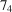International Tables for Crystallography (2016). Vol. A, ch. 3.2, pp. 720-776https://doi.org/10.1107/97809553602060000930

## Contents

• 3.2. Point groups and crystal classes  (pp. 720-776)
• 3.2.1. Crystallographic and noncrystallographic point groups  (pp. 720-737) | html | pdf |
• 3.2.1.1. Introduction and definitions  (pp. 720-721) | html | pdf |
• 3.2.1.2. Crystallographic point groups  (pp. 721-731) | html | pdf |
• 3.2.1.2.1. Description of point groups  (pp. 721-722) | html | pdf |
• 3.2.1.2.2. Crystal and point forms  (pp. 722-727) | html | pdf |
• 3.2.1.2.3. Description of crystal and point forms  (pp. 727-729) | html | pdf |
• 3.2.1.2.4. Notes on crystal and point forms  (pp. 729-730) | html | pdf |
• 3.2.1.2.5. Names and symbols of the crystal classes  (pp. 730-731) | html | pdf |
• 3.2.1.3. Subgroups and supergroups of the crystallographic point groups  (p. 731) | html | pdf |
• 3.2.1.4. Noncrystallographic point groups  (pp. 731-737) | html | pdf |
• 3.2.1.4.1. Description of general point groups  (pp. 731-733) | html | pdf |
• 3.2.1.4.2. The two icosahedral groups  (pp. 733-735) | html | pdf |
• 3.2.1.4.3. Sub- and supergroups of the general point groups  (pp. 735-737) | html | pdf |
• 3.2.2. Point-group symmetry and physical properties of crystals  (pp. 737-741) | html | pdf |
• 3.2.2.1. General restrictions on physical properties imposed by symmetry  (pp. 737-739) | html | pdf |
• 3.2.2.1.1. Neumann's principle  (p. 737) | html | pdf |
• 3.2.2.1.2. Curie's principle  (pp. 737-738) | html | pdf |
• 3.2.2.1.3. Enantiomorphism, enantiomerism, chirality, dissymmetry  (pp. 738-739) | html | pdf |
• 3.2.2.1.4. Polar directions, polar axes, polar point groups  (p. 739) | html | pdf |
• 3.2.2.2. Morphology  (pp. 739-740) | html | pdf |
• 3.2.2.3. Etch figures  (p. 740) | html | pdf |
• 3.2.2.4. Optical properties  (pp. 740-741) | html | pdf |
• 3.2.2.4.1. Refraction  (p. 740) | html | pdf |
• 3.2.2.4.2. Optical activity  (pp. 740-741) | html | pdf |
• 3.2.2.4.3. Second-harmonic generation (SHG)  (p. 741) | html | pdf |
• 3.2.2.5. Pyroelectricity and ferroelectricity  (p. 741) | html | pdf |
• 3.2.2.6. Piezoelectricity  (p. 741) | html | pdf |
• 3.2.3. Tables of the crystallographic point-group types  (pp. 742-771) | html | pdf |
• 3.2.4. Molecular symmetry  (pp. 772-776) | html | pdf |
• 3.2.4.1. Introduction  (p. 772) | html | pdf |
• 3.2.4.2. Definitions  (pp. 772-773) | html | pdf |
• 3.2.4.3. Tables of the point groups  (pp. 773-774) | html | pdf |
• 3.2.4.4. Polymeric molecules  (pp. 774-775) | html | pdf |
• 3.2.4.5. Enantiomorphism and chirality  (pp. 775-776) | html | pdf |
• References | html | pdf |
• Figures
• Fig. 3.2.1.1. The 47 crystal forms that crystals may take  (p. 727) | html | pdf |
• Fig. 3.2.1.2. Maximal subgroups and minimal supergroups of the two-dimensional crystallographic point groups  (p. 731) | html | pdf |
• Fig. 3.2.1.3. Maximal subgroups and minimal supergroups of the three-dimensional crystallographic point groups  (p. 732) | html | pdf |
• Fig. 3.2.1.4. Subgroups and supergroups of the two-dimensional general point groups  (p. 735) | html | pdf |
• Fig. 3.2.1.5. The subgroups of the two-dimensional general point groups 16mm (4N-gonal system) and 18mm [-gonal system, including the-gonal groups]  (p. 735) | html | pdf |
• Fig. 3.2.1.6. Subgroups and supergroups of the three-dimensional general point groups  (p. 736) | html | pdf |
• Fig. 3.2.4.1. P-helix of isotactic poly-4-methyl-1-pentene (form I) with a 7/2 helix which corresponds to ahelix in Hermann–Mauguin notation  (p. 774) | html | pdf |
• Tables
• Table 3.2.1.1. The ten two-dimensional crystallographic point groups, arranged according to crystal system  (p. 720) | html | pdf |
• Table 3.2.1.2. The 32 three-dimensional crystallographic point groups, arranged according to crystal system (cf. Chapter 2.1)  (p. 721) | html | pdf |
• Table 3.2.1.3. The 47 crystallographic face and point forms, their names, eigensymmetries, and their occurrence in the crystallographic point groups (generating point groups)  (pp. 724-726) | html | pdf |
• Table 3.2.1.4. Names and symbols of the 32 crystal classes  (p. 728) | html | pdf |
• Table 3.2.1.5. Classes of general point groups in two dimensions (N = integer0)  (p. 733) | html | pdf |
• Table 3.2.1.6. Classes of general point groups in three dimensions (N = integer0)  (p. 734) | html | pdf |
• Table 3.2.2.1. The 11 Laue classes, the 21 noncentrosymmetric crystallographic point groups (crystal classes) and the occurrence (+) of specific crystal properties  (p. 738) | html | pdf |
• Table 3.2.2.2. Polar axes and nonpolar directions in the 21 noncentrosymmetric crystal classes  (p. 739) | html | pdf |
• Table 3.2.2.3. Categories of crystal systems distinguished according to the different forms of the indicatrix  (p. 740) | html | pdf |
• Table 3.2.3.1. The ten two-dimensional crystallographic point groups  (pp. 742-744) | html | pdf |
• Table 3.2.3.2. The 32 three-dimensional crystallographic point groups  (pp. 745-769) | html | pdf |
• Table 3.2.3.3. The two icosahedral point groups  (pp. 770-771) | html | pdf |
• Table 3.2.4.1. Classes of rod groups  (p. 775) | html | pdf |